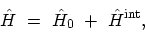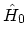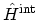# B. Time Evolution Pictures

The time evolution of operators and state vectors in quantum mechanics can be expressed in different representations. The SCHRÖDINGER, the interaction, and the HEISENBERG representations are useful in analyzing the second-quantized form of the SCHRÖDINGER equation. The HAMILTONian is assumed to be of the form (see (3.1))(B.1)

whereis the non-interacting part, which is assumed to be exactly solvable.contains all the interactions, such as carrier-carrier, carrier-phonon, impurity scattering, and so forth.
Subsections M. Pourfath: Numerical Study of Quantum Transport in Carbon Nanotube-Based Transistors